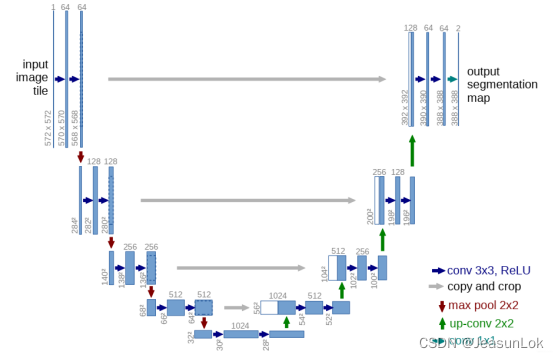1.UNet整体结构理解

1.1 UNet结构拆解

1.1.1 卷积层主体：两次卷积操作

1.1.2 左部分每一层：下采样+卷积层

1.1.3 右部分每一层：上采样+中部分跳跃连接+卷积层

1.1.4 输入层和输出层

1.2 UNet结构融合

2.UNet Pytorch代码理解

2.1 UNet基本组件编码

2.1.1 卷积层编码

2.1.2 左部分层编码（下采样+卷积层）

2.1.3 右部分层编码（上采样+跳跃连接+卷积层）

2.1.4 输出层编码（输出结果采用1*1卷积）

2.2 UNet整体网络编码

# 1.UNet整体结构理解

关于UNet的介绍网上有很多，它在语义分割上的传奇地位是任何深度学习初学者在接触CNN时都一定会知晓的。因而在此就不再赘述一些网络特点优势等等等等，我们直接来理解网络的组成和结构就好了。

先放上UNet用得烂了但也被称作“存在一大批魔改”的结构图：在这里，我们可以把UNet拆解成三个部分进行理解：左、右、中三部分。

• 左：特征提取模块，主要操作是进行下采样（池化）
• 右：特征融合模块，主要操作是进行上采样
• 中：跳跃连接模块，主要操作是将左右相同层两个模块的特征连接起来

上述就是整个UNet结构的主要理解，理解完成之后，我们将各个模块进行编码上的拆解，之后再融合起来，组成整个UNet的结构。

## 1.1 UNet结构拆解

### 1.1.1 卷积层主体：两次卷积操作

在卷积层内，卷积之后得到的特征图进行BatchNorm操作，再进入激活函数ReLU即可。所以这就是我们第一个需要编码的小模块。

### 1.1.2 左部分每一层：下采样+卷积层

这部分就比较简单了，除了最后一层之外，所有都需要进行下采样，所以结合1.1.1中的卷积层，每一层做一次kerner_size=2的下采样+卷积层（两次卷积操作）即可。

### 1.1.3 右部分每一层：上采样+中部分跳跃连接+卷积层

这里需要结合中部分和右部分的网络结构来看，对于右部分每一层最终得到的特征图而言，其应该是下一层上采样之后，结合左部分相同层特征图的跳跃连接，再经过卷积层（两次卷积操作）得到的。所以对应操作应该是先做一次scale_factor=2的上采样，并将之前相同层数得到的下采样结果做一个cat连接，再经过卷积层。

### 1.1.4 输入层和输出层

由于输入层无需下采样且输出层无需上采样，所以输入层和输出层都只需要经过一层卷积层（两次卷积操作）即可。

## 1.2 UNet结构融合

所以综合来看，整个UNet模型应该是：

左边输入层的in_channels是你所用图片的层数，右边输出层的out_channels是你所需要做分割的类别个数。其他的所有参数参照上面的UNet结构图即可。

# 2.UNet Pytorch代码理解

## 2.1 UNet基本组件编码

### 2.1.1 卷积层编码

``````class DoubleConv(nn.Module):
"""(convolution => [BN] => ReLU) * 2"""

def __init__(self, in_channels, out_channels):
super().__init__()
self.double_conv = nn.Sequential(
nn.BatchNorm2d(out_channels),
nn.ReLU(inplace=True),
nn.BatchNorm2d(out_channels),
nn.ReLU(inplace=True)
)

def forward(self, x):
return self.double_conv(x)``````

可以看到整个卷积层的主体部分就是（Conv2d->BatchNorm2d->ReLU），没什么好说的。

卷积层类命名为DoubleConv，意为做两次卷积。

### 2.1.2 左部分层编码（下采样+卷积层）

``````class Down(nn.Module):
"""Downscaling with maxpool then double conv"""

def __init__(self, in_channels, out_channels):
super().__init__()
self.maxpool_conv = nn.Sequential(
nn.MaxPool2d(2),
DoubleConv(in_channels, out_channels)
)

def forward(self, x):
return self.maxpool_conv(x)
``````

基于2.1.1的卷积层，左部分层的编码也非常简单，直接就是（MaxPool2d->DoubleConv）。

左部分层类命名为Down，意为下采样，但下采样在编码的时候也做了两次卷积。

### 2.1.3 右部分层编码（上采样+跳跃连接+卷积层）

``````class Up(nn.Module):
"""Upscaling then double conv"""

def __init__(self, in_channels, out_channels, bilinear=True):
super().__init__()

# if bilinear, use the normal convolutions to reduce the number of channels
if bilinear:
self.up = nn.Upsample(scale_factor=2, mode='bilinear', align_corners=True)
else:
self.up = nn.ConvTranspose2d(in_channels // 2, in_channels // 2, kernel_size=2, stride=2)# //为整数除法

self.conv = DoubleConv(in_channels, out_channels)

def forward(self, x1, x2):
x1 = self.up(x1)
# input is CHW
diffY = torch.tensor([x2.size() - x1.size()])
diffX = torch.tensor([x2.size() - x1.size()])

x1 = F.pad(x1, [diffX // 2, diffX - diffX // 2,
diffY // 2, diffY - diffY // 2])

x = torch.cat([x2, x1], dim=1)
return self.conv(x)``````

这一层理解起来会稍微复杂一点，但总体结构是不变的，还是上采样+跳跃连接+卷积层

首先是bilinear的理解，从代码上可以看出来，用与不用bilinear会导致不同策略的上采样。使用bilinear会采用Upsample的上采样，其算法为插值上采样，不需要训练参数，速度比较快；不使用bilinear则会采用ConvTranspose2d的上采样，其算法是逆卷积化，需要训练参数，所以速度会比较慢，但上采样的数据会比较好。具体Upsample和ConvTranspose2d的参数可以查阅Pytorch官网或者网上的说明。

其次是卷积操作conv，直接调用DoubleConv即可，不再赘述。

最后看forward前向传播的操作里，可以看到在前向传播过程中，是先做了up操作，然后再做cat操作，最后return了conv即做了卷积操作。所以这也符合我们上面的说法。

input是tensor即CHW，（channels,height,width），但是初看x1.size()真的很奇怪，这不对吧。后来查了查，发现是（batchsize, channel, height, width），所以x2.size()-x1.size()是张量x2和张量x1的行差，x2.size()-x1.size()是张量x2和张量x1的列差。

``````torch.nn.functional.pad(input, pad, mode='constant', value=0)
``````

最后再采用torch.cat函数将同层左部分特征图与右部分上采样后特征图在dim=1即行维度上进行拼接，这就完成了跳跃连接。

### 2.1.4 输出层编码（输出结果采用1*1卷积）

``````class OutConv(nn.Module):
def __init__(self, in_channels, out_channels):
super(OutConv, self).__init__()
self.conv = nn.Conv2d(in_channels, out_channels, kernel_size=1)

def forward(self, x):
return self.conv(x)``````

最后输出层则是需要直接进行一个卷积层（两次卷积）操作，其两次卷积均采用1*1的卷积核得到最终的输出结果（语义分割类别）。

## 2.2 UNet整体网络编码

完成2.1四个基本组件的编码后，即可得到以下UNet整体的网络编码：其通道数与其他参数如UNet的结构图所示，其前向传播forward的组成结构严格按照1.2的顺序

``````class UNet(nn.Module):
def __init__(self, n_channels, n_classes, bilinear=True):
super(UNet, self).__init__()
self.n_channels = n_channels # 输入通道数
self.n_classes = n_classes # 输出类别数
self.bilinear = bilinear # 上采样方式

self.inc = DoubleConv(n_channels, 64) # 输入层
self.down1 = Down(64, 128)
self.down2 = Down(128, 256)
self.down3 = Down(256, 512)
self.down4 = Down(512, 512)
self.up1 = Up(1024, 256, bilinear)
self.up2 = Up(512, 128, bilinear)
self.up3 = Up(256, 64, bilinear)
self.up4 = Up(128, 64, bilinear)
self.outc = OutConv(64, n_classes) # 输出层

def forward(self, x):
x1 = self.inc(x) # 一开始输入
x2 = self.down1(x1) # 四层左部分
x3 = self.down2(x2)
x4 = self.down3(x3)
x5 = self.down4(x4)
x = self.up1(x5, x4) # 四层右部分
x = self.up2(x, x3)
x = self.up3(x, x2)
x = self.up4(x, x1)
logits = self.outc(x) # 最终输出
return logits``````